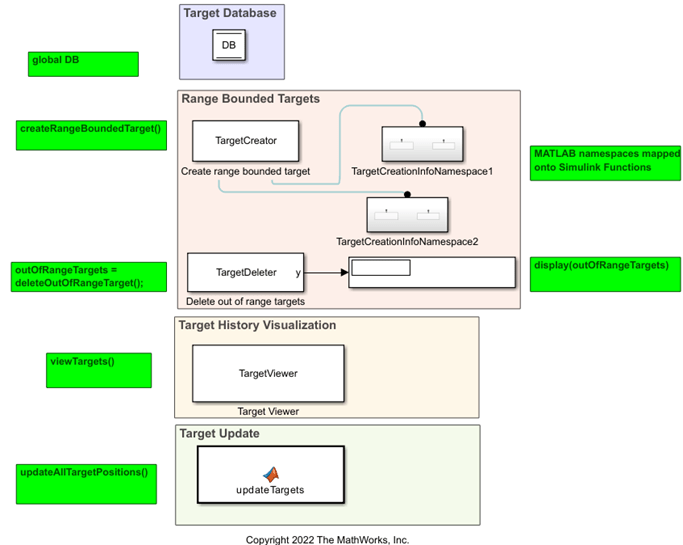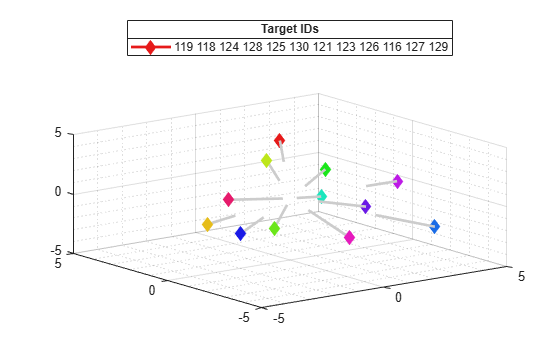## Model Moving Target Visualization by Converting MATLAB Code to Simulink Behavior

This topic shows how to convert MATLAB® code to a Simulink® model. First, an example shows how to convert a MATLAB algorithm for moving target visualization to a Simulink model with the same functionality. Then, a table shows common conversions from MATLAB code to Simulink behavior.

### Convert Moving Target MATLAB Code to a Simulink Model

This example shows you how to convert MATLAB algorithms to a Simulink model using a moving target example. The conversion allows you to build models containing MATLAB and Simulink components. This example uses MATLAB code for target creation and deletion then shows you how to convert that functionality into Simulink.

Moving Target MATLAB Code

Open Script

This example uses MATLAB to create, move, visualize, and delete targets. Two different MATLAB packages generate two separate target databases. The target creator is a System object® since it requires the use of states. The target database is a global database. The target checker, which determines if the target is out of range and deletes the target if it is, is a System object because it requires the use of states. The target position updater is a simple algorithm and is therefore a MATLAB function. The target visualizer is a System object and requires the use of states.

For this example, an `initDB` function is used to initialize the global database and create the Target Struct. Then, the targets are created using the `Target Creator function` and the `Target Creation info Package`. The out-of-range targets are then deleted. The target range is 5 for this example, so any target greater than 5 is deleted, and the remaining target positions are updated. This process is looped over 100 times and visualized as it runs.Open this modelThe MATLAB script can be converted into a Simulink model that has the same functionality. The `Target Database` is converted to a DSM using the `Model PostLoadFcn` and `Simulink.Bus.createObject`. Convert the `Target Creation info Package` to a Simulink Function. The target creator, target deleter, and target viewer are each converted into a MATLAB System block since they use System objects in MATLAB. The target viewer also uses a Display block to visualize the targets as the simulation runs. The target updater is converted into a MATLAB Function block since it is a simple MATLAB function that does not use System objects or states. The number of time steps in the loop corresponds with the stop time in the toolbar.

The target creator uses scoped functions in the MATLAB code to call the target creation packages. Use the `getGlobalNamesImpl` and `getSimulinkFunctionNamesImpl` APIs in Simulink to declare the function names for the `Target Creator` System object. These functions declare the target creation package position and velocity names in the target creation package so the package can be called by the System objects in Simulink.

To run the model, click `Run`. After the model compiles, it produces a visualization just like in the MATLAB code.### Common Conversions of MATLAB Code to Simulink Behavior

The following table shows some cases of MATLAB code mapping to Simulink blocks and functions. Note: this list is not comprehensive.

`struct`Bus
Array of `struct`Array of buses
GlobalDSM
FunctionMATLAB Function block
System objectMATLAB System block
System object calls to external functionsSystem block calls to Simulink functions
Scheduling algorithmStateflow® chart
Top-level loop (for example: ```"for t=1:0.1:10"```)Simulink start time, stop time, and time step
Parameter tuning in MATLAB algorithmsSimulink tunable parameters
Iteration over an arrayFor-each subsystem
`if-else` loopIf block and if-action subsystem
Switch-case statementSwitch Case block and Switch Case Action Subsystem block
`while` loopWhile Iterator block
Global resource access (for example: database, file handle, communication port, hardware device)DSM or Simulink functions
Persistent variablesDiscrete states in System object or "persistent" keyword in MATLAB Function block
Dynamic arrayVariable-size (bounded now, unbounded in future)
Fixed-point `fi` objectSimulink fixed-point
Cell arrayUse `struct` to represent uniform cell array